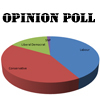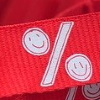#### You may also like### Mathland Election

A political commentator summed up an election result. Given that there were just four candidates and that the figures quoted were exact find the number of votes polled for each candidate.### Matching Fractions, Decimals and Percentages

Can you match pairs of fractions, decimals and percentages, and beat your previous scores?### Fractions and Percentages Card Game

Can you find the pairs that represent the same amount of money?

# Put Out the Flags

##### Age 11 to 14Challenge Level

Aditya sent us a very well explained solution to this problem:

Tim has $50$% blue, $35$% red, $10$% white and $5$% union jacks.
Beth has $40$% blue, $32$% red, $20$% white and $8$% union jacks.

In fractions, this is:

T = $\frac{1}{2}$ blue, $\frac{7}{20}$red, $\frac{1}{10}$ white & $\frac{1}{20}$ union jack.
= $\frac{10}{20}$ blue, $\frac{7}{20}$ red, $\frac{2}{20}$ white and $\frac{1}{20}$ union jacks.
Therefore Tim has $20$ flags
.
B = $\frac{2}{5}$ blue, $\frac{8}{25}$ red, $\frac{1}{5}$ white & 2/25 $\frac{2}{25}$ union jacks.
= $\frac{10}{25}$ blue, $\frac{8}{25}$ red, $\frac{5}{25}$ white and $\frac{2}{25}$ union jacks.
Therefore Beth has $25$ flags
.

Now, we know that Beth has more flags than Tim. Beth has one more red flag, and both have the same number of blue flags. Between them, they have $3$ union jacks.

The second part of the problem:
Out of every $20$ flags Tim would have $1$ union jack.
Out of every $25$ flags Beth would have $2$ union jacks.
So first we thought about how many different ways you could make $10$ union jacks
 Tim Beth 8 2 6 4 4 6 2 8

For each of these we then need toknow the total number of flags :
 Tim Beth Tim has this number of flags Beth has this number of flags Total number of flags 8 2 160 25 185 6 4 120 50 170 4 6 80 75 155 2 8 40 100 140
That is assuming that they both have some flags!